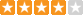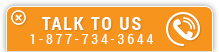# Brayton Cycle (Gas Turbine) Ideal vs Real Operation for Power Application Analysis

Course Number: M-1036
Credit: 1 PDH
Subject Matter Expert: Gordan Feric, P.E.
Price: \$29.95 Purchase using Reward Tokens. Details6 reviews
Overview

#### In Brayton Cycle (Gas Turbine) Ideal vs Real Operation for Power Application Analysis, you'll learn ...

• Understand basic energy conversion engineering assumptions and equations
• Know basic components of the Brayton Cycle (Gas Turbine) and its T - s diagram
• Be familiar with the Brayton Cycle ideal and real operation
• Understand general Brayton Cycle performance trends

#### OverviewPreview a portion of this course before purchasing it.

Credit: 1 PDH

Length: 24 pages

In this one hour course, the open, simple Brayton Cycle used for stationary power generation is considered.

The Brayton Cycle thermal efficiency is presented only for the air as the working fluid. The thermal efficiency derivation is presented with a simple mathematical approach. The Brayton Cycle is presented in a T - s diagram and its major performance trends (thermal efficiency, specific power output and power output) are plotted in a few figures as a function of compression ratio, gas turbine inlet temperature, working fluid mass flow rate and both isentropic compression and expansion efficiency. It should be noted that this online course does not deal with costs (capital, operational or maintenance).

In this course, the student gets familiar with the Brayton Cycle, its components, T - s diagram, ideal and real operation and major performance trends.

#### Specific Knowledge or Skill Obtained

This course teaches the following specific knowledge and skills:

• Understand basic energy conversion engineering assumptions and equations
• Know basic components of the Brayton Cycle (Gas Turbine) and its T - s diagram
• Be familiar with the Brayton Cycle ideal and real operation
• Understand general Brayton Cycle performance trends

#### Certificate of Completion

You will be able to immediately print a certificate of completion after passing a multiple-choice quiz consisting of 10 questions. PDH credits are not awarded until the course is completed and quiz is passed.

Board Acceptance
 This course is applicable to professional engineers in: Alabama (P.E.) Alaska (P.E.) Arkansas (P.E.) Delaware (P.E.) Florida (P.E. Area of Practice) Georgia (P.E.) Idaho (P.E.) Illinois (P.E.) Illinois (S.E.) Indiana (P.E.) Iowa (P.E.) Kansas (P.E.) Kentucky (P.E.) Louisiana (P.E.) Maine (P.E.) Maryland (P.E.) Michigan (P.E.) Minnesota (P.E.) Mississippi (P.E.) Missouri (P.E.) Montana (P.E.) Nebraska (P.E.) Nevada (P.E.) New Hampshire (P.E.) New Jersey (P.E.) New Mexico (P.E.) New York (P.E.) North Carolina (P.E.) North Dakota (P.E.) Ohio (P.E. Self-Paced) Oklahoma (P.E.) Oregon (P.E.) Pennsylvania (P.E.) South Carolina (P.E.) South Dakota (P.E.) Tennessee (P.E.) Texas (P.E.) Utah (P.E.) Vermont (P.E.) Virginia (P.E.) West Virginia (P.E.) Wisconsin (P.E.) Wyoming (P.E.)
Reviews (6)
More DetailsPreview a portion of this course before purchasing it.

Credit: 1 PDH

Length: 24 pages